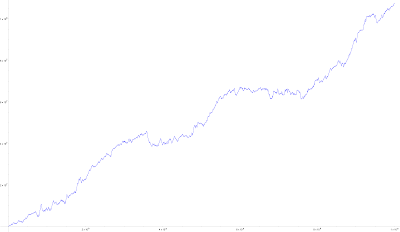## Tuesday, October 24, 2023

Éric Angelini's latest offering is based on the absolute differences (AD, be they odd or even) of an integer sequence's adjacent digits determining the sequence's new numbers. After Giorgos Kalogeropoulos posted one million terms, I struggled to better his (apparently Mathematica) result to five million terms before realizing that my one-at-a-time AppendTo approach was not the best tool for this game.

Starting with 315 terms of the sequence (s) we generate — in twelve steps — over one billion terms by repetitively determining the adjacent-digits sequence, creating therefrom an absolute differences sequence, and applying a sign change to those differences that are even. Prepend zero, Accumulate, add 10.

Table[d=Flatten[IntegerDigits[s]]; c=Abs[Most[d]-Rest[d]]; f=Prepend[Table[If[OddQ[c[[i]]],c[[i]],-c[[i]]], {i,Length[c]}], 0]; s=Accumulate[f]+10; Length[s], {12}]

{577, 1181, 2719, 6976, 19556, 57525, 203635, 791890, 3733165, 21270116, 140287834, 1002951921}

That's it! Truncate the final 1002951921 terms to 10^9 terms, take every tenth integer (Mathematica has trouble enough plotting 100 million points), and graph:click to enlarge